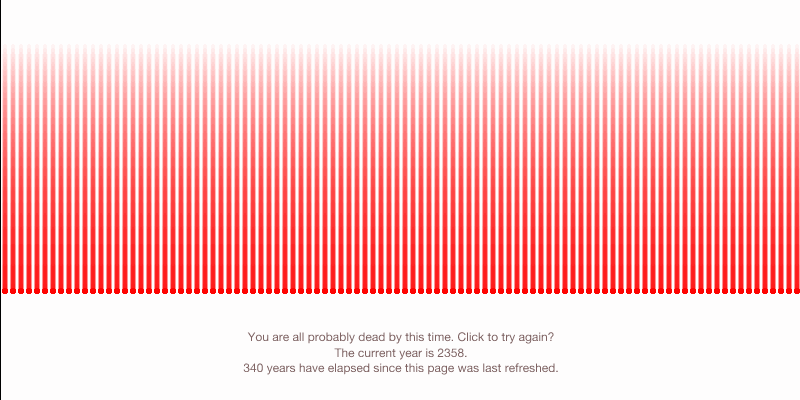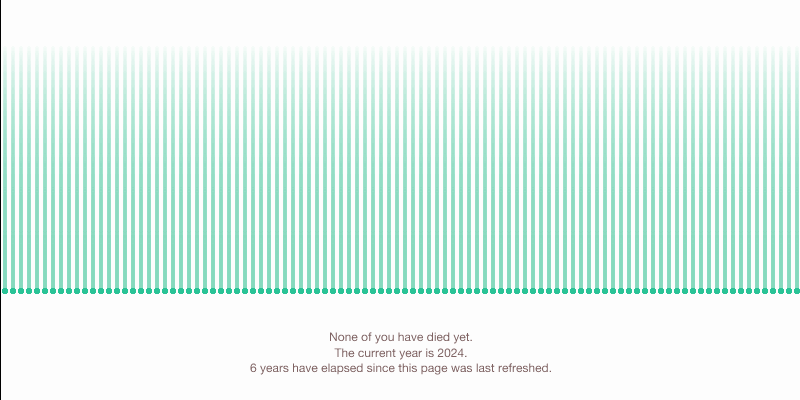# zbeok-ClockTime is often valuable only due to its scarcity. In our case, we only have as much as our lifespan allows. My clock measures time in micromorts, which is a unit that is one out of a million chance of death. When this clock runs in real time, it takes 100 “yous” and assigns them each an amount of micromorts. Then, for every unit of time (in my case milliseconds) the clock performs a check of which people have died. Done in real time, the clock mostly doesn’t seem to be doing anything; however, over the course of years, one may see that the “yous” slowly die off one by one, and a person running this clock will essentially have to race the clock to emerge alive. (Above within the gifs, this process is replaced with a timelapse at the timing of one year a second.)

Doing this project was more of a conceptual struggle than a technical one. I think that there might have been more of an effort in emphasizing the totality of death: perhaps if this program were more final or somehow embedded within another more permanent system than p5.js. In addition, I could have emphasized the humanity of the data points on the screen, making the tension more apparent. However, I do like the cold look of this format in the sense that deaths are often just data points. Micromorts and statistics are detached numbers. Overall, I’m pretty satisfied with my concept.```// Simple p5.js Clock Template // Golan Levin, 2016   var prevSec; var millisRolloverTime; var w = 800; var h = 400; var meLim = 100; var mes = []; var fader = h*5/8; var USMMs = 22; var fps = 60; var USMMperms = USMMs/(24*60*60*1000*fps*1000000000); // var USMMperms = USMMs *365/ (fps*1000000000); var sWeight = 5; var ease = new p5.Ease(); var deaths = 0; var initMs = 0; var initY = 0; var txt = "None of you have died yet.";   function preload() { myFont = loadFont('http://localhost:8000/AlteHaasGroteskRegular.ttf'); } //--------------------------   function setup() { textFont(myFont); frameRate(fps); createCanvas(800, 400); millisRolloverTime = 0; init(); }   function init() { mes = []; deaths=0; makeYou(); initMs = millis(); initY = year(); }   function mouseClicked() { init(); console.log('wow'); txt = "None of you have died yet."; } //-------------------------- function draw() { background(255); // My favorite pink   // Fetch the current time var H = hour(); var M = minute(); var S = second(); E = (millis()-initMs)/1000;   // Reckon the current millisecond, // particularly if the second has rolled over. // Note that this is more correct than using millis()%1000; if (prevSec != S) { millisRolloverTime = millis(); } prevSec = S; var mils = floor(millis() - millisRolloverTime); noStroke(); fill(128,100,100); // text("Hour: " + H, 10, 22); // text("Minute: " + M, 10, 42); // text("Second: " + S, 10, 62); // text((E), 100, 62); // text("Millis: " + mils, 10, 82); textAlign(CENTER); text(txt, w/2, h*7/8); var Y = year()-initY; text("The current year is "+ year()+ ".\n"+round(Y)+" years have elapsed since this page was last refreshed.", w/2, h*7/8 + 16);   var secondsWithFraction = S + (mils / 1000.0); var secondBarWidthSmooth = map(secondsWithFraction, 0, 60, 0, width);   noStroke(); // fill(255, 219, 240); // rect(0, 250, secondBarWidthSmooth, 20); drawTrail(E); if (deaths&gt;=meLim-1) { txt = "You are all probably dead by this time. Click to try again?" } } function makeYou() { for (var i=0;i&lt;meLim;i++) { var n = mes.length+1; var me = {start:millis(), mms:USMMperms, x:w/meLim/2+(i*w/meLim), y:(h*3/4) , num: i, dead:false}; mes.push(me); } }   function drawTrail(S) { for (var i=0;i&lt;fader;i++) { // checkStats(mes[i]); for (var j=0;j&lt;meLim;j++) { // console.log(i); isItDead(mes[j],S); if (mes[j].dead) { stroke(255,0,0,100-i/fader*100); point(mes[j].x,mes[j].y-i); point(mes[j].x,mes[j].y);   } else { strokeWeight(sWeight-1); stroke(135, 219, 190,200-i/fader*200); point(mes[j].x,mes[j].y-i);   strokeWeight(sWeight); stroke(45, 196, 150); point(mes[j].x,mes[j].y); } } } } // function checkStats(me) { // isItDead(me); // } function isItDead(me,S) { if (me.dead == true) { return; } var rand = random(0,1); if (rand&lt;me.mms) { deaths++; me.dead=true; console.log("The "+(me.num+1)+"th you is dead, for a total of "+deaths+" deaths! Alas!"); txt = (deaths+" out of 100 yous have died! Alas."); } var p = ease["cubicIn"](S*USMMperms); me.mms = randomGaussian(me.mms+p,USMMperms); }```